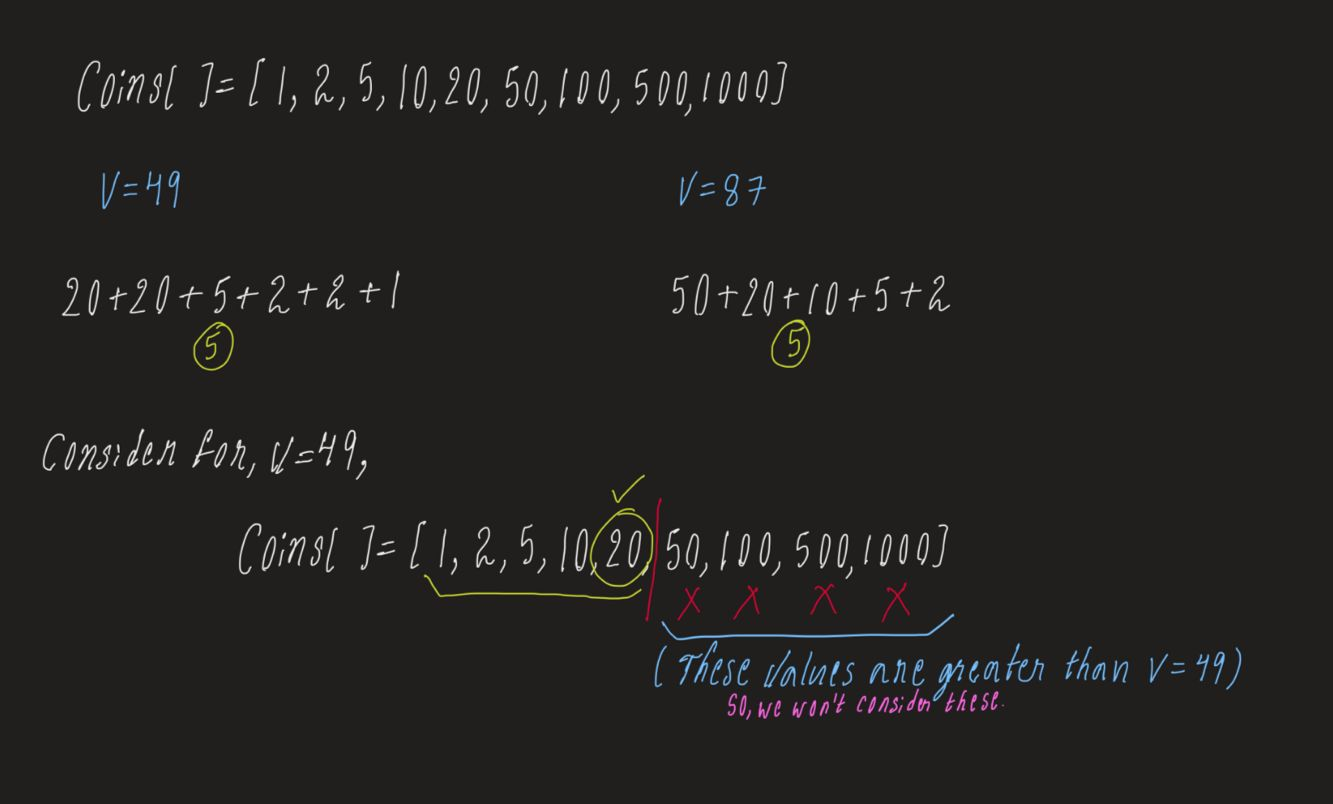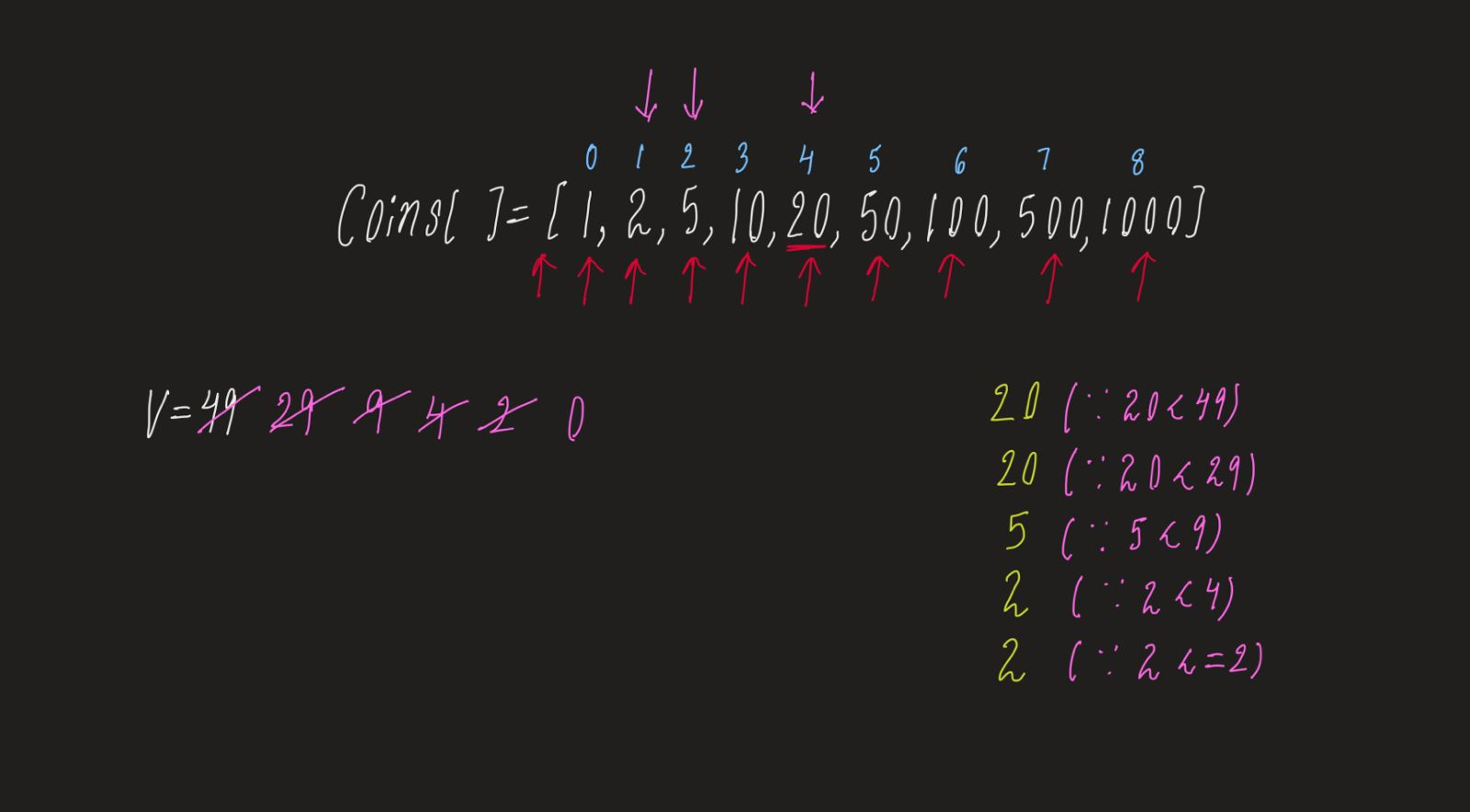# Find minimum number of coins

Problem Statement: Given a value V, if we want to make a change for V Rs, and we have an infinite supply of each of the denominations in Indian currency, i.e., we have an infinite supply of { 1, 2, 5, 10, 20, 50, 100, 500, 1000} valued coins/notes, what is the minimum number of coins and/or notes needed to make the change.

Examples:

```Example 1:

Input: V = 70

Output: 2

Explaination: We need a 50 Rs note and a 20 Rs note.

Example 2:

Input: V = 121

Output: 3

Explaination: We need a 100 Rs note, a 20 Rs note and a 1 Rs coin.
```

Solution:

Disclaimer: Don’t jump directly to the solution, try it out yourself first.

Solution: Greedy Algorithm

Approach: We will keep a pointer at the end of the array i. Now while(V >= coins[i]) we will reduce V by coins[i] and add it to the ans array.

We will also ignore the coins which are greater than V and the coins which are less than V. We consider them and reduce the value of V by coins[I].Code:

## C++ Code

``````
#include<bits/stdc++.h>

using namespace std;
int main() {
int V = 49;
vector < int > ans;
int coins[] = {1, 2, 5, 10, 20, 50, 100, 500, 1000};
int n = 9;
for (int i = n - 1; i >= 0; i--) {
while (V >= coins[i]) {
V -= coins[i];
ans.push_back(coins[i]);
}
}
cout<<"The minimum number of coins is "<<ans.size()<<endl;
cout<<"The coins are "<<endl;
for (int i = 0; i < ans.size(); i++) {
cout << ans[i] << " ";
}

return 0;
}
``````

Output:

The minimum number of coins is 5
The coins are
20 20 5 2 2

Time Complexity:O(V)

Space Complexity:O(1)

## Java Code

``````
import java.util.*;
public class Main {
public static void main(String[] args) {

int V = 49;
ArrayList < Integer > ans = new ArrayList < > ();
int coins[] = {1, 2, 5, 10, 20, 50, 100, 500, 1000};
int n = coins.length;
for (int i = n - 1; i >= 0; i--) {
while (V >= coins[i]) {
V -= coins[i];
}
}
System.out.println("The minimum number of coins is "+ans.size());
System.out.println("The coins are ");
for (int i = 0; i < ans.size(); i++) {
System.out.print(" " + ans.get(i));
}

}
}
``````

Output:

The minimum number of coins is 5
The coins are
20 20 5 2 2

Time Complexity:O(V)

Space Complexity:O(1)

Special thanks to P.C.Bhuvaneshwari for contributing to this article on takeUforward. If you also wish to share your knowledge with the takeUforward fam, please check out this article